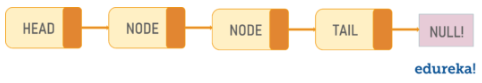AWS Global Infrastructure

### Programming & Frameworks

Topics Covered
• C Programming and Data Structures (17 Blogs)
• Comprehensive Java Course (4 Blogs)
• Java/J2EE and SOA (345 Blogs)
• Spring Framework (8 Blogs)
SEE MORE

# All you Need to Know About List Function in PHPFunctions in PHP are of great importance, especially the one which control the flow of items. So, in this article, we will discuss List Function in PHP in the following order:

## What is List Function?

The list function in PHP is an inbuilt function which is used to assign the array values to multiple variables at a time while execution. Like array (), this is not really a function, but a language construct. list() is used to assign a list of variables in one operation.This function will only work on numerical arrays. When the user assigns multiple values to the array, then the first element in the array is the first variable, the second one will be the second variable and so on, till the number of variables is there.

The number of variables cannot exceed the length of the numerical array if it exceeds it gives error.parameter types and return types are not written. A function with no return statements implicitly returns NULL.

Syntax:

`list(\$variable1, \$variable2....)`

## Parameters of the List Function in PHP

The list function in PHP parameter will accept a list of variables separated by spaces in code. A parameter is just like a variable. To add more functionality to a function, we can add parameters.parameters are specified after the function name, inside the parentheses. These variables are assigned values by the user. The first variable is mandatory to the user.

 Name Description Required/ Optional Type \$variable1 The first variable to be assigned Required Mixed* \$variable2..n Next variable to be assigned Optional Mixed*

* Mixed: Mixed indicates that a parameter may accept multiple (but not necessary all) types.

Return Value: The function returns the assigned array to the multiple variables passed by the user. It does not assign a value to the \$variable m if m>n, where n is the length of the array.

Now let’s have a look at different examples for list functions in PHP.

## Examples of List() Function

Example 1:

```<?php
\$array = array(1, 2, 3, 4);
list(\$a, \$b, \$c) = \$array;
echo "x =", (\$a), "n";
echo " y =", (\$b), "n";
echo " z =", (\$c), "n";
echo "x+y-c =", (\$a*\$b*\$c);
?>
```

Output:

x=1
y =2
z =3
x+y-z =0

Example 2:

```<?php
\$w3r1_array = array(“php”, “javascript”, “asp”);
list( \$x, \$y, \$z) = \$w3r1_array;
echo “We have covered \$x, \$y and \$z. “;
\$w3r2_array = array(“php”, “javascript”, “asp”);
list(\$x, \$z) = \$w3r2_array;
echo “We have covered \$x and \$z and so many other topics”;
?>
```

Output:

`We have covered php, javascript and asp. We have covered php and asp and so many other topics`

With this, we come to an end of this List Function in PHP article. I hope you got an understanding of what exactly happens here.

Check out the PHP Certification Training by Edureka, a trusted online learning company with a network of more than 250,000 satisfied learners spread across the globe.

Got a question for us? Please mention it in the comments section of ”List Function in PHP” and I will get back to you.REGISTER FOR FREE WEBINARThank you for registering Join Edureka Meetup community for 100+ Free Webinars each month